Home > CCA2 > Chapter 8 > Lesson 8.3.1 > Problem8-121

8-121.

Now Carlos needs to solve 2x3 + 3x2 − 8x + 3 = 0, but his calculator will still only create a standard graph. He sees that the graph of y = 2x3 + 3x2 − 8x + 3 crosses the x-axis at x = 1. Find all three solutions to the equation. Homework Help ✎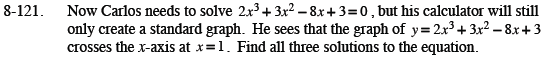If x = 1, then x − 1 = 0.
So divide 2x3 + 3x2 − 8x + 3 = 0 by x − 1.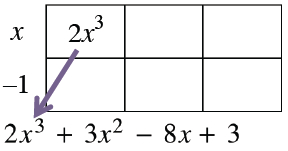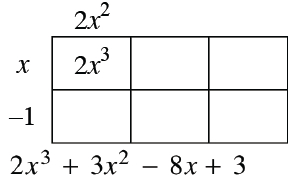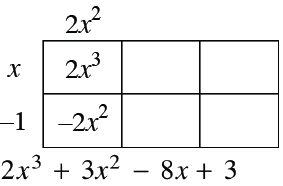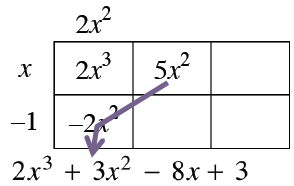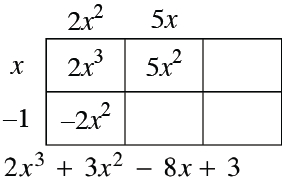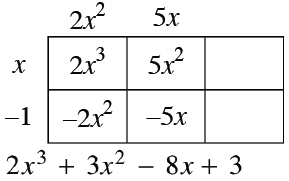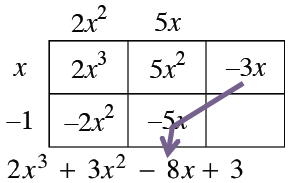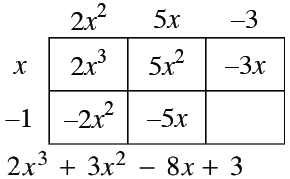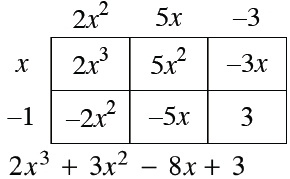Write the equation in factored form.
(x − 1)(2x2 + 5x − 3) = 0

Factor the quadratic expression and use the Zero Product Property to obtain the three solutions.